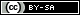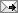## Gavin R. Putland,  BE PhD

 Monday, February 02, 2015 (Comment)

# Fine control over sizes of fractions in LaTeX

LATEX provides two styles for fractions: \displaystyle, which has full-sized characters and is the default for displayed equations, and \textstyle, which has smaller characters and is the default for in-text equations. But it often happens that \displaystyle seems too big, e.g. because it over-emphasizes the fraction or because space is tight, while \textstyle is unnecessarily small. Hence I have found it useful to define three new fraction commands with intermediate sizes. From largest to smallest, they are \sdfrac, which uses \small characters with \displaystyle; \fdfrac, which uses \footnotesize characters with \displaystyle; and \ltfrac, which sets the character size to \large but then reduces it by using \textstyle. The definitions are as follows:

\newcommand{\sdfrac}{\mbox{\small$\displaystyle\frac{#1}{#2}$}}
\newcommand{\fdfrac}{\mbox{\footnotesize$\displaystyle\frac{#1}{#2}$}}
\newcommand{\ltfrac}{\mbox{\large$\frac{#1}{#2}$}}

Because the character-sizing commands do not work in math mode, each definition first switches to text mode using \mbox, then changes the size, then switches back to math mode (and \textstyle) using the ‘\$’ delimiters. While \fdfrac and \ltfrac produce similar-sized characters, the latter has less space under non-descending characters in the numerator, and less space around plus and minus signs.

Also note that the amsmath package provides \tfrac, which forces \textstyle, and \dfrac, which forces \displaystyle. All of the above commands are as easy to use as the standard \frac, but give more control over size.TweetReturn to Contents